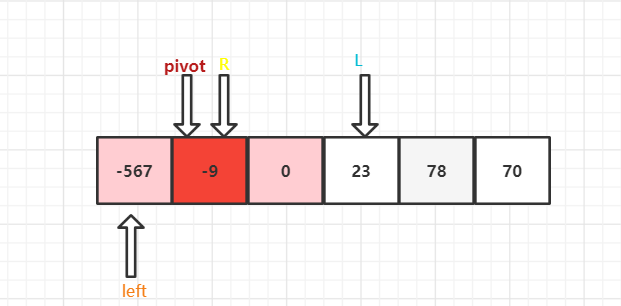﻿ java 排序算法之快速排序_java_脚本之家
java# java 排序算法之快速排序

## 基本思想

• (1)首先设定一个分界值（基准值），通过该分界值将数组分成左右两部分。
• (2)将大于或等于分界值的数据集中到数组右边，小于分界值的数据集中到数组的左边。此时，左边部分中各元素都小于或等于分界值，而右边部分中各元素都大于或等于分界值。
• (3)然后，左边和右边的数据可以独立排序。对于左侧的数组数据，又可以取一个分界值，将该部分数据分成左右两部分，同样在左边放置较小值，右边放置较大值。右侧的数组数据也可以做类似处理。
• (4)重复上述过程，可以看出，这是一个递归定义。通过递归将左侧部分排好序后，再递归排好右侧部分的顺序。当左、右两个部分各数据排序完成后，整个数组的排序也就完成了。• 上图以 最后一个元素的值 作为基准
• 比基准值小的，排在左侧，比基准值大的排在右侧
• 然后再以分好的部分重复以上操作，直到每个部分中只有一个数据时，就排好序了

## 思路分析

• 原始数组：`arr = [-9,78,0,23,-567-70]`• 设置两个变量，左下标`L = 0`，右下标`R = 数组大小 - 1`，选数组中间的值为基准值，`pivot = arr[(L + R )/ 2]` ,基准值为 0。再用两个变量保存 左下标 和 右下标，`left = L``right = R`，用于后面的排序做准备。• 左边一组，从左到右，挨个与 基准值 比较，找出比基准值大的值，跳出查找循环• 右边一组，从右到左，挨个与 基准值 比较，找出比基准值小的值，跳出查找循环• 可以看到左右两组各找到一个对应的值，那么就让他们进行交换• 然后继续找，直到左右两边碰到，• `L``R` 分别前进一步和后退一步，• 先对左边的组进行快速排序，同样进行上面的操作，• 因为这里 `left` 直接就指向了 `pivot` ，所以不用进行移动查找了。`R``pivot`进行比较 ，-567 < -9 ，`R``left`进行交换，得到如下图，注：`pivot` 是一个值,不是引用类型• 右边的组也是同样的道理，这里就不作过多的解析了```l ------------ pivot --------------- r

```

## 代码实现

### 推导实现

```    @Test
public void processDemo() {
int arr[] = {-9, 78, 0, 23, -567, 70};
System.out.println("原始数组：" + Arrays.toString(arr));
processQuickSort(arr, 0, arr.length - 1);
}

/**
* @param arr
* @param left  左边这一组的下标起始点，到中间值，则为一组
* @param right 右边这一组的下标结束点，到中间值，则为一组
*/
public void processQuickSort(int[] arr, int left, int right) {
/*
基本思想：选择一个基准值，将基准值小分成一组，比基准值大的分成一组。
这里的实现思路：
1. 挑选的基准值为数组中间的值
2. 中间值就把数组分成了两组
3. 左边一组，从左到右，挨个与 基准值 比较，找出比基准值大的值
4. 右边一组，从右到左，挨个与 基准值 比较，找出比基准值小的值
5. 左右两边各找到一个值，那么就让这两个值进行交换
6. 然后继续找，直到左右两边碰到，这一轮就结束。这一轮就称为快速排序
7. 继续对分出来的小组，进行上述的快速排序操作，直到组内只剩下一个数时，则排序完成

l ------------ pivot --------------- r
一组从左往右找               一组从右往左找
*/
int l = left;
int r = right;
// 中心点，让这个点作为基准值
int pivot = arr[(left + right) / 2];
// 当他们没有碰到的时候，说明还这一轮还可以继续找
while (l < r) {
// 左边组：当找到大于基准值时，退出循环，则表示该值需要交换到右侧去: arr[l] > pivot
//      也就是说，如果 arr[l] < pivot，则表示还没有找到比基准值大的数
//      注意：不能等于 pivort，因为最差的情况没有找到，则最后 arr[l] 就是 pivot 这个值，也同样退出循环
while (arr[l] < pivot) {
l++; // 所以让左边这一组继续找
}

// 右边组：当找到小于基准值时，退出循环，则表示该值需要交换到左侧去：arr[r] < pivot
//      也就是说，如果 arr[l] > pivot，则表示还没有找到比基准值小的数
//注意：这里也同样跟上面一样，不能等于 pivort
while (arr[r] > pivot) {
r--;//让右边组继续找
}

// 当左侧与右侧相碰时，说明两边都没有找到，这一轮不用进行交换
// 等于表示，找到了中间的下标
if (l >= r) {
break;
}

// 当找到时，则两数进行交换。
//注意：这里可能会出现有一组已经找完了，或者没有找到，但是另一组找到了，所以一个是指向 pivot 的，
//另一个则是指向要交换的数,交换后 pivot的值在数组中的位置会发生改变，下次的交换方式就会发生变化。这个地方要动脑筋想想。
int temp = arr[l];
arr[l] = arr[r];
arr[r] = temp;

// 当交换后，
// 当数组是： {-9, 78, 0, -23, 0, 70}  时（pivot的值在数组中有多个），就可以验证这里的逻辑
// 如果没有这个判定，将会导致，l 永远 小于 r。循环不能退出来的情况，就出现死循环了
if (arr[l] == pivot) {
/*
l 自己不能往前移动 一步，因为当交换完成后为：{-9, 0, 0, -23, 78, 70}
l = 1,arr[l] = 0
r = 4,arr[r] = 78
再经过一次循环后
l = 1,arr[l] = 0
r = 3,arr[r] = -23
交换后数组为：{-9，-23，0，0，78，70}
此时 l = 1，arr[l] = -23;r = 3,arr[r] = 0
又经过一次循环后
l = 2,arr[l] = 0
r = 3,arr[r] = 0
数组为：{-9，-23，0，0，78，70}
进入了死循环
这里好好动脑子想想

这里为什么是用r-=1呢？是因为if里面的条件是arr[l] == pivot，如果要排序的数组中不存在多个和基准值相等的值，
那么用l+=1的话，l就会跑过分界线（基准值），跑到另一组去，这个算法也就失败了，
还有一个原因是，r 是刚刚交换过的，一定比 基准值大，所以没有必要再和基准值比较了
*/
r -= 1;
}
// 这里和上面一致，如果说，先走了上面的 r-=1
// 这里也满足（也就是说有多个值和基准值相等），那么说明，下一次是相同的两个值，一个是 r == 一个是基准值
// 但是他们是相同的值，r后退一步 l前进一步，不影响。但是再走这完这里逻辑时，就会导致  l > r，退出整个循环
if (arr[r] == pivot) {
l += 1;
}
}
System.out.println("第 1 轮排序后：" + Arrays.toString(arr));
}
```

```        System.out.println("第 1 轮排序后：" + Arrays.toString(arr));

/*
if (l >= r) {
break;
}
循环从这条代码中跳出就会l = r
*/
// 如果 l = r，会出现死循环，出现栈溢出
//这里也要动脑子想想
if (l == r) {
l++;
r--;
}

// 开始左递归
// 上面算法是 r--，l++ ，往两组的中间走，当 left < r 时，表示还可以继续分组
if (left < r) {
processQuickSort(arr, left, r);
}

if (right > l) {
processQuickSort(arr, l, right);
}
```

### 完整实现

```  /**
* 快速排序默写实现
*
*     基本思想：通过一趟将要排序的数据，分隔成独立的两个部分，一部分的所有数据都比另一部分的所有数据要小。
*     思路分析：
*      {-9, 78, 0, 23, -567, 70};  length=6
*      1. 挑选中间的值作为 基准值：(0 + (6 -1))/2=  = 0
*      2. 左侧 left 部分，从 0 开始到中间值 -1： 0,1: -9, 78,找出一个比基准值大的数
*      3. 右侧 right 部分，从中间值 + 1 到数组大小-1：3,5：23,-567, 70，找出一个比基准值小的数
*      4. 如果找到，则将他们进行交换，这样一轮下来，就完成了一次快速排序：一部分的所有数据都比另一部分的所有数据要小。
*      4. 如果左侧部分还可以分组，则进行左侧递归调用
*      5. 如果右侧部分还可以分组，则进行右侧递归调用
*
*    简单说：一轮快速排序示意图如下：
*                   中间的基准值
*      l ------------ pivot --------------- r
*      一组从左往右找               一组从右往左找
*      找到比基准值大的数          找出一个比基准值小的数
*                    然后进行交换
*
*/
@Test
public void quickSortTest() {
int arr[] = {-9, 78, 0, 23, -567, 70};
//        int arr[] = {-9, 78, 0, -23, 0, 70}; // 在推导过程中，将会导致交换异常的数组，在这里不会出现那种情况
int left = 0;
int right = arr.length - 1;
System.out.println("原始数组：" + Arrays.toString(arr));
quickSort(arr, left, right);
System.out.println("排序后：" + Arrays.toString(arr));
}

public void quickSort(int[] arr, int left, int right) {
// 找到中间值
int pivotIndex = (left + right) / 2;
int pivot = arr[pivotIndex];
int l = left;
int r = right;
while (l < r) {
// 从左往右找，直到找到一个数，比基准值大的数
while (arr[l] < pivot) {
l++;
}
// 从右往左找，知道找到一个数，比基准值小的数
while (arr[r] > pivot) {
r--;
}
// 表示未找到
if (l >= r) {
break;
}
// 进行交换
int temp = arr[l];
arr[l] = arr[r];
arr[r] = temp;

// 那么下一轮，左侧的这个值将不再参与排序，因为刚交换过，一定比基准值小
// 那么下一轮，右侧的这个值将不再参与排序，因为刚交换过，一定比基准值大
r--;
l++;
}

// 当一轮找完后，没有找到，则是中间值时，
// 需要让他们擦肩而过，也就是重新分组，中间值不再参与分组
// 否则，在某些情况下，会进入死循环
if (l == r) {
l++;
r--;
}
// 如果左侧还可以继续分组，则继续快排
// 由于擦肩而过了，那么左侧的组值，则是最初的开始与中间值的前一个，也就是这里得到的 r
if (left < r) {
quickSort(arr, left, r);
}
if (right > l) {
quickSort(arr, l, right);
}
}
```

## 大数据量耗时测试

```    /**
* 大量数据排序时间测试
*/
@Test
public void bulkDataSort() {
int max = 80000;
//        int max = 8;
int[] arr = new int[max];
for (int i = 0; i < max; i++) {
arr[i] = (int) (Math.random() * 80000);
}
if (arr.length < 10) {
System.out.println("原始数组：" + Arrays.toString(arr));
}
Instant startTime = Instant.now();
//        processQuickSort(arr, 0, arr.length - 1);  // 和老师的原版代码对比，结果是一样的
quickSort(arr, 0, arr.length - 1);
if (arr.length < 10) {
System.out.println("排序后：" + Arrays.toString(arr));
}
Instant endTime = Instant.now();
System.out.println("共耗时：" + Duration.between(startTime, endTime).toMillis() + " 毫秒");
}
```